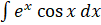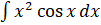### INTEGRATION 2

Mathematics SSS 3 Second Term

WEEK 2

INTEGRATION 2

Performance Objectives

Student should be able to:

1. Solve problems of integration using: integration by part method.
2. Solve problems on integration using: integration by partial fraction method.
3. Solve problems involving definite integral.
4. Apply integration to find the area under the curve or between a curve.

Content

Integration by Part Method

This method has been introduced in week 1 hence we would treat a few more examples to further deepen our understanding of the method.

Example 1: Evaluate the following using the integration by part method

1.ii.Solution